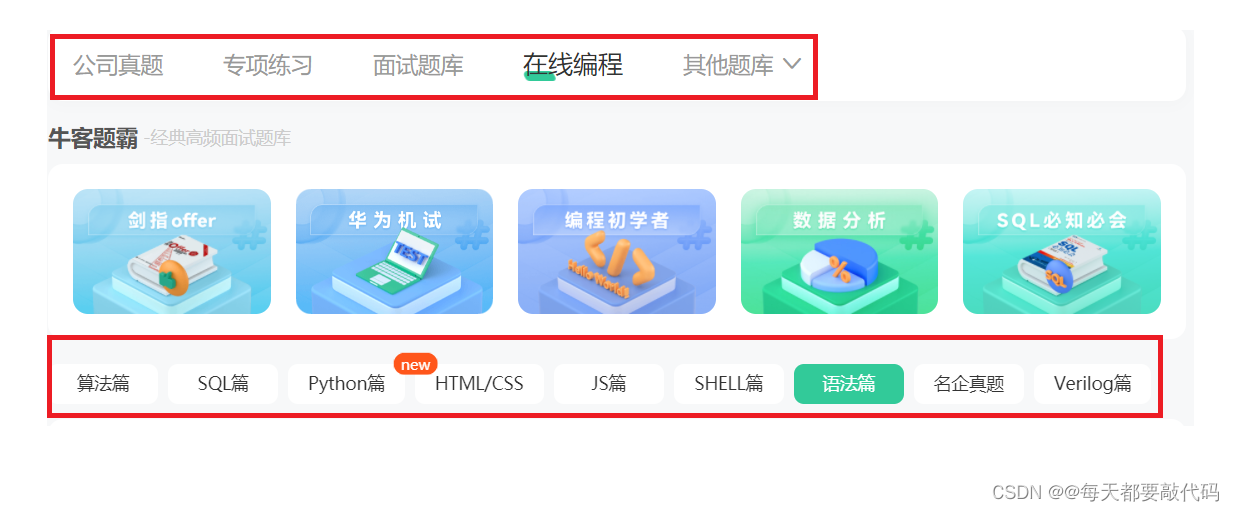> 文档中心 > 带你刷（牛客网）C语言百题（第三天）

# 带你刷（牛客网）C语言百题（第三天）

✅作者简介：大家好我是@每天都要敲代码，一位材料转码农的选手，希望一起努力，一起进步！
📃个人主页：@每天都要敲代码的个人主页
🔥系列专栏：牛客网C语言刷题专栏
💬推荐一款模拟面试、刷题神器，从基础到大厂面试题👉点击跳转刷题网站进行注册学习# 习题一：出生日期输入输出_牛客题霸_牛客网

year=2013
month=02
date=25

``#include int main(){    // 方法1：看成三个数    int y=0,m=0,d=0;    scanf("%4d%2d%2d",&y,&m,&d);    //指定带宽    printf("year=%4d\nmonth=%02d\ndate=%02d\n",y,m,d); //方法2：看成一个数    int date = 0;    scanf("%d",&date);    printf("year=%4d\nmonth=%02d\ndate=%02d\n",date/10000,date/100%100,date%100);    return 0;}``

# 习题二：按照格式输入并交换输出_牛客题霸_牛客网

``#include int main(){ int a,b;    scanf("a=%d,b=%d",&a,&b);    // 方法1：借用临时变量    int tmp = a;    a = b;    b = tmp;    printf("a=%d,b=%d",a,b); // 方法2：使用+，有可能溢出    a = a+b;    b = a-b;    a = a-b;    printf("a=%d,b=%d",a,b); // 方法3：使用异或^    a = a^b;    b = a^b;    a = a^b;    printf("a=%d,b=%d",a,b); return 0;}``

# 习题三：大小写转换_牛客题霸_牛客网

A
B

a
b

``#include #include int main(){    // 1.使用库函数tolower    int ch = 0;    while((ch = getchar()) != EOF){ //多组输入，一般都是写成这个格式 printf("%c",tolower(ch)); //使用tolower库函数    }    // 2.不使用库函数    while((ch = getchar()) != EOF){putchar(ch+32); //大写和小写差32,例如：A是65，a是97getchar(); //吸收遗留的\nprintf("\n"); //换行    }    return 0;}``

# 习题四：十六进制转十进制_牛客题霸_牛客网

BoBo写了一个十六进制整数ABCDEF，他问KiKi对应的十进制整数是多少。

printf可以使用使用格式控制串“%md”输出域宽为m的十进制整数。

``#includeint main(){    printf("%15d\n",0XABCDEF); //十六进制数是以0X开头的    return 0;}``

# 习题五：缩短二进制_牛客题霸_牛客网

printf可以使用使用格式控制串“%o”、“%X”分别输出八进制整数和十六进制整数，并使用修饰符“#”控制前导显示

``#include int main(){    // 输入十进制数    int n = 1234;    scanf("%d",&n);    // 打对应的八进制和十六进制    printf("%#o %#X",n,n); //#在输出打印的时候会显示八进制前面的0，和十六进制前面的0X}``

# 习题六：牛牛的空格分隔_牛客题霸_牛客网

a

1

1.23

a 1 1.230000

``#include int main(){    char c;    int i;    float f;    scanf("%c\n%d\n%f\n",&c,&i,&f);    printf("%c %d %.6f",c,i,f);    return 0;}``

# 结束语

💬刷题神器，从基础到大厂面试题👉点击跳转刷题网站